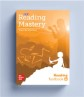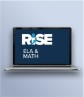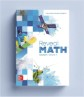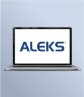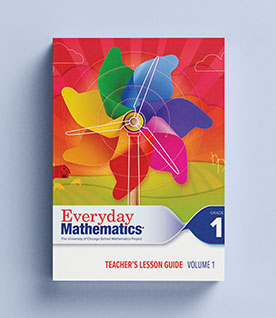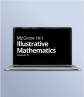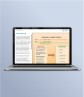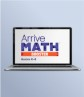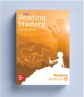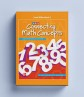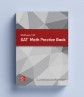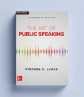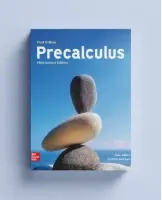## Our Principles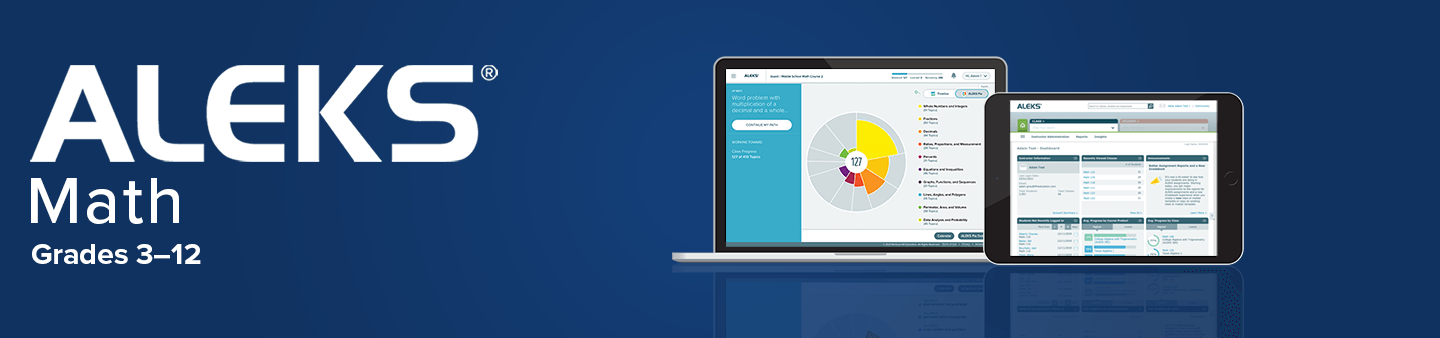# ALEKS Math for Grades 3–12​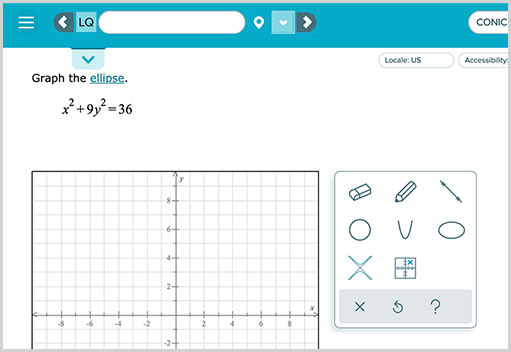ALEKS is an online math assessment and adaptive learning program for grades 3–12 that helps students review and successfully master the skills needed to meet critical mathematical benchmarks and standards. Using adaptive questioning, ALEKS precisely identifies which math concepts a student knows and doesn’t know within a course. Then, through individualized instruction, each student is provided with a personalized learning pathway that focuses on the exact topics they are most ready to learn, allowing ALEKS to effectively target any unfinished learning while minimizing frustration, accelerating momentum, and building confidence.

Using an automatic cycle of assessment, ALEKS ensures individualized learning pathways are continually refreshed based on each student’s unique needs. These assessments also provide instructors with visibility at a granular level with reports measuring progress by student, topic, or standard.

ALEKS course content spans from Grade 3 to Precalculus which allows students to work on lesson-level content at their own pace while still acquiring prerequisite skill support or extending their learning, making it ideal for remediation, intervention, or enrichment settings.## Focus and Accelerate Learning with New Priority Standards Courses

With new Priority Standards Courses for Grades 3–5 and Algebra 1, teachers have the option of having students focus exclusively on prerequisite topics and grade-level standards that will be critical to their success within the full grade-level course. These courses allow teachers to address unfinished learning and accelerate students to grade level while still supporting them with the individualized Learning Paths that ALEKS is known for providing. When students master at least 75 percent of the topics within the Priority Standards Course, teachers can confidently move them into the standard grade-level course to learn and master the remaining content for that grade.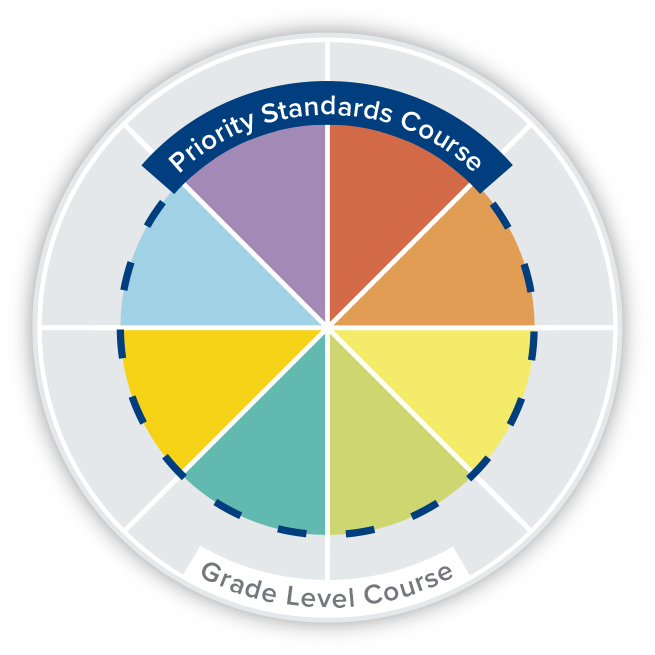ALEKS Math 3–12 Courses

(EN-SP) Fully Bilingual Course - English and Spanish

## Elementary

Course Curriculum:

ALEKS QuickTables is a research-based, math fact mastery program for multiplication, division, addition, and subtraction for grades 3 and up. The online program offers ongoing assessment, learning, review, and visual tracking of student progress.

46 of 95 total topics are included in this priorities-focused course for students who may need additional support for unfinished learning. Once students have mastered 75 percent of these topics, they can be moved to the full grade-level course.

Course Curriculum:

• Whole Numbers
• Fractions
• Decimals
• Measurement and Geometry
• Other Topics Available(*)

View Full Course Curriculum

The complete ALEKS course for third grade.

Course Curriculum:

• Whole Numbers
• Fractions
• Decimals
• Measurement and Geometry
• Other Topics Available(*)

View Full Course Curriculum

98 of 194 total topics are included in this priorities-focused course for students who may need additional support for unfinished learning. Once students have mastered 75 percent of these topics, they can be moved to the full grade-level course.

Course Curriculum:

• Whole Numbers
• Fractions
• Decimals
• Measurement and Geometry
• Other Topics Available(*)

View Full Course Curriculum

The complete ALEKS course for fourth grade.

Course Curriculum:

• Whole Numbers
• Fractions
• Decimals
• Measurement and Geometry
• Other Topics Available(*)

View Full Course Curriculum

163 of 261 total topics are included in this priorities-focused course for students who may need additional support for unfinished learning. Once students have mastered 75 percent of these topics, they can be moved to the full grade-level course.

Course Curriculum:

• Whole Numbers
• Fractions
• Decimals
• Measurement and Geometry
• Proportions and Percents
• Signed Numbers and Exponents
• Other Topics Available(*)

View Full Course Curriculum

The complete ALEKS course for fifth grade.

Course Curriculum:

• Whole Numbers
• Fractions
• Decimals
• Measurement and Geometry
• Proportions and Percents
• Signed Numbers and Exponents
• Other Topics Available(*)

View Full Course Curriculum

Course Curriculum:

• Whole Numbers
• Fractions
• Decimals
• Measurement and Geometry
• Proportions and Percents
• Signed Numbers and Exponents
• Other Topics Available(*)

View Full Course Curriculum

Course Curriculum:

• Whole Number Place Value
• Subtracting Whole Numbers
• Multiplying Whole Numbers
• Dividing Whole Numbers
• Fractions
• Measurement
• Representing and Interpreting Data
• Perimeter and Area
• Geometry
• Other Topics Available(*)

View Full Course Curriculum

Course Curriculum:

• Whole Number Place Value, Addition, and Subtraction
• Multiplying Whole Numbers
• Dividing Whole Numbers
• Expressions and Equations
• Fraction Equivalence
• Operations with Fractions
• Fractions and Decimals
• Measurement
• Perimeter and Area
• Geometry
• Other Topics Available(*)

View Full Course Curriculum

Course Curriculum:

• Place Value and Review of Arithmetic
• Multiplying Whole Numbers
• Dividing Whole Numbers
• Multiplying and Dividing Decimals
• Fractions
• Operations with Fractions
• Expressions and Equations
• Measurement
• Geometry
• Perimeter, Area, and Volume
• Other Topics Available(*)

View Full Course Curriculum

Course Curriculum:

• Whole Numbers
• Decimals
• Fractions
• Ratios, Proportions, and Measurement
• Percents
• Integers and Rational Numbers
• Equations and Inequalities
• Graphs and Functions
• Lines, Angles, and Polygons
• Transformations
• Perimeter, Area, and Volume
• Data Analysis and Probability
• Other Topics Available(*)

View Full Course Curriculum

## Middle School

Course Curriculum:

• Whole Numbers
• Decimals
• Fractions
• Ratios, Proportions, and Measurement
• Percents
• Integers and Rational Numbers
• Equations and Inequalities
• Graphs and Functions
• Lines, Angles, and Polygons
• Transformations
• Perimeter, Area, and Volume
• Data Analysis and Probability
• Other Topics Available(*)

View Full Course Curriculum

Course Curriculum:

• Whole Numbers and Integers
• Fractions
• Decimals
• Ratios, Proportions, and Measurement
• Percents
• Equations and Inequalities
• Graphs, Functions, and Sequences
• Lines, Angles, and Polygons
• Perimeter, Area, and Volume
• Data Analysis and Probability
• Other Topics Available(*)

View Full Course Curriculum

Course Curriculum:

• Whole Numbers and Integers
• Fractions
• Decimals
• Ratios, Proportions, and Measurement
• Percents
• Equations and Inequalities
• Graphs, Functions, and Sequences
• Lines, Angles, and Polygons
• Transformations
• Perimeter, Area, and Volume
• Data Analysis and Probability
• Other Topics Available(*)

View Full Course Curriculum

Course Curriculum:

• Whole Numbers and Integers
• Fractions
• Decimals
• Ratios, Proportions, and Measurement
• Percents
• Equations and Inequalities
• Graphs, Functions, and Sequences
• Perimeter, Area, and Volume
• Data Analysis and Probability
• Other Topics Available(*)

View Full Course Curriculum

Course Curriculum:

• Whole Numbers and Integers
• Fractions
• Decimals
• Ratios, Proportions, and Measurement
• Percents
• Equations and Inequalities
• Graphs, Functions, and Sequences
• Lines, Angles, and Polygons
• Transformations
• Perimeter, Area, and Volume
• Data Analysis and Probability
• Other Topics Available(*)

View Full Course Curriculum

Course Curriculum:

• Whole Numbers and Integers
• Fractions
• Decimals
• Ratios, Proportions, and Measurement
• Percents
• Equations and Inequalities
• Graphs, Functions, and Sequences
• Lines, Angles, and Polygons
• Transformations
• Perimeter, Area, and Volume
• Data Analysis and Probability
• Other Topics Available(*)

View Full Course Curriculum

Course Curriculum:

• Real Numbers
• Linear Equations
• Linear Inequalities
• Functions and Lines
• Linear Systems
• Data Analysis and Probability
• Other Topics Available(*)

View Full Course Curriculum

Course Curriculum:

• Real Numbers
• Linear Equations
• Linear Inequalities
• Functions and Lines
• Linear Systems
• Exponents and Exponential Functions
• Polynomials and Factoring
• Other Topics Available(*)

View Full Course Curriculum

Course Curriculum:

• Real Numbers
• Linear Equations
• Linear Inequalities
• Functions and Lines
• Linear Systems
• Data Analysis and Probability
• Other Topics Available(*)

View Full Course Curriculum

Course Curriculum:

• Real Numbers
• Linear Equations
• Linear Inequalities
• Functions and Lines
• Linear Systems
• Exponents and Exponential Functions
• Polynomials and Factoring
• Other Topics Available(*)

View Full Course Curriculum

397 of 494 total topics are included in this priorities-focused course for students who may need additional support for unfinished learning. Once students have mastered 75 percent of these topics, they can be moved to the full grade-level course.

Course Curriculum:

• Real Numbers
• Linear Equations
• Linear Inequalities
• Functions and Lines
• Linear Systems
• Exponents and Exponential Functions
• Polynomials and Factoring
• Data Analysis and Probability
• Other Topics Available(*)

View Full Course Curriculum

The complete ALEKS course for Algebra 1.

Course Curriculum:

• Real Numbers
• Linear Equations
• Linear Inequalities
• Functions and Lines
• Linear Systems
• Exponents and Exponential Functions
• Polynomials and Factoring
• Data Analysis and Probability
• Other Topics Available(*)

View Full Course Curriculum

Course Curriculum:

• Real Numbers
• Linear Equations
• Linear Inequalities
• Functions and Lines
• Linear Systems
• Exponents and Exponential Functions
• Polynomials and Factoring
• Data Analysis and Probability
• Other Topics Available(*)

View Full Course Curriculum

Course Curriculum:

• Whole Numbers
• Decimals
• Fractions
• Ratios, Proportions, and Measurement
• Percents
• Integers and Rational Numbers
• Equations and Inequalities
• Graphs and Functions
• Lines, Angles, and Polygons
• Transformations
• Perimeter, Area, and Volume
• Data Analysis and Probability
• Other Topics Available(*)

View Full Course Curriculum

Course Curriculum:

• Whole Numbers
• Fractions
• Decimals
• Geometry
• Measurement and Graphs
• Proportions, Percents, and Probability
• Algebra
• Other Topic Available(*)

View Full Course Curriculum

Course Curriculum:

• Whole Numbers
• Fractions
• Decimals
• Geometry
• Measurement and Graphs
• Proportions, Percents, and Probability
• Algebra
• Other Topics Available(*)

View Full Course Curriculum

Course Curriculum:

• Whole Numbers
• Fractions
• Decimals
• Geometry
• Measurement and Graphs
• Proportions, Percents, and Probability
• Algebra
• Other Topics Available(*)

View Full Course Curriculum

Course Curriculum:

• Whole Numbers
• Fractions
• Decimals
• Geometry
• Measurement and Data
• Algebra
• Other Topics Available(*)

View Full Course Curriculum

Course Curriculum:

• Whole Numbers
• Decimals
• Fractions
• Lines, Angles, and Polygons
• Perimeter, Area, and Volume
• Data Analysis and Probability
• Other Topics Available(*)

View Full Course Curriculum

Course Curriculum:

• Whole Numbers and Integers
• Fractions
• Decimals
• Ratios, Proportions, and Measurement
• Percents
• Graphs, Functions, and Sequences
• Lines, Angles, and Polygons
• Perimeter, Area, and Volume
• Data Analysis and Probability
• Other Topics Available(*)

View Full Course Curriculum

## High School

Course Curriculum:

• Whole Numbers and Integers
• Fractions
• Decimals
• Ratios, Proportions, and Measurement
• Percents
• Equations and Inequalities
• Graphs, Functions, and Sequences
• Perimeter, Area, and Volume
• Data Analysis and Probability
• Other Topics Available(*)

View Full Course Curriculum

Course Curriculum:

• Whole Numbers and Integers
• Fractions
• Decimals
• Ratios, Proportions, and Measurement
• Percents
• Equations and Inequalities
• Graphs, Functions, and Sequences
• Lines, Angles, and Polygons
• Transformations
• Perimeter, Area, and Volume
• Data Analysis and Probability
• Other Topics Available(*)

View Full Course Curriculum

Course Curriculum:

• Whole Numbers and Integers
• Fractions
• Decimals
• Ratios, Proportions, and Measurement
• Percents
• Equations and Inequalities
• Graphs, Functions, and Sequences
• Lines, Angles, and Polygons
• Transformations
• Perimeter, Area, and Volume
• Data Analysis and Probability
• Other Topics Available(*)

View Full Course Curriculum

Course Curriculum:

• Whole Numbers and Integers
• Fractions
• Decimals
• Ratios, Proportions, and Measurement
• Percents
• Equations and Inequalities
• Graphs, Functions, and Sequences
• Lines, Angles, and Polygons
• Transformations
• Perimeter, Area, and Volume
• Data Analysis and Probability
• Other Topics Available(*)

View Full Course Curriculum

Course Curriculum:

• Whole Numbers
• Fractions
• Decimals
• Ratios, Percents, and Measurement
• Real Numbers
• Equations and Inequalities
• Graphing
• Exponents and Polynomials
• Geometry and Data Analysis
• Other Topics Available(*)

View Full Course Curriculum

Course Curriculum:

• Real Numbers
• Linear Equations
• Linear Inequalities
• Functions and Lines
• Linear Systems
• Data Analysis and Probability
• Other Topics Available(*)

View Full Course Curriculum

Course Curriculum:

• Real Numbers
• Linear Equations
• Linear Inequalities
• Functions and Lines
• Linear Systems
• Data Analysis and Probability
• Other Topics Available(*)

View Full Course Curriculum

Course Curriculum:

• Real Numbers
• Linear Equations
• Linear Inequalities
• Functions and Lines
• Linear Systems
• Exponents and Exponential Functions
• Polynomials and Factoring
• Other Topics Available(*)

View Full Course Curriculum

Course Curriculum:

• Real Numbers
• Linear Equations
• Linear Inequalities
• Functions and Lines
• Linear Systems
• Exponents and Exponential Functions
• Polynomials and Factoring
• Other Topics Available(*)

View Full Course Curriculum

397 of 494 total topics are included in this priorities-focused course for students who may need additional support for unfinished learning. Once students have mastered 75 percent of these topics, they can be moved to the full grade-level course.

Course Curriculum:

• Real Numbers
• Linear Equations
• Linear Inequalities
• Functions and Lines
• Linear Systems
• Exponents and Exponential Functions
• Polynomials and Factoring
• Data Analysis and Probability
• Other Topics Available(*)

View Full Course Curriculum

The complete ALEKS course for Algebra 1.

Course Curriculum:

• Real Numbers
• Linear Equations
• Linear Inequalities
• Functions and Lines
• Linear Systems
• Exponents and Exponential Functions
• Polynomials and Factoring
• Data Analysis and Probability
• Other Topics Available(*)

View Full Course Curriculum

Course Curriculum:

• Whole Numbers
• Fractions
• Decimals and Percents
• Real Numbers
• Linear Equations
• Linear Inequalities
• Functions and Lines
• Systems of Linear Equations and Inequalities
• Exponents and Exponential Functions
• Polynomials and Factoring
• Data Analysis and Probability
• Other Topics Available(*)

View Full Course Curriculum

Course Curriculum:

• Real Numbers
• Linear Equations
• Linear Inequalities
• Functions and Lines
• Linear Systems
• Exponents and Exponential Functions
• Polynomials and Factoring
• Data Analysis and Probability
• Other Topics Available(*)

View Full Course Curriculum

Course Curriculum:

• Real Numbers and Algebraic Expressions
• Linear Equations
• Linear Inequalities
• Lines and Functions
• Systems
• Exponents
• Polynomials and Factoring
• Other Topics Available(*)

View Full Course Curriculum

Course Curriculum:

• Arithmetic and Algebra Review
• Segments and Angles
• Reasoning
• Lines
• Triangles
• Similarity
• Right Triangles and Trigonometry
• Transformations
• Area and Volume
• Circles
• Probability and Data Analysis
• Other Topics Available(*)

View Full Course Curriculum

Course Curriculum:

• Real Numbers
• Linear Equations and Inequalities
• Graphs and Functions
• Linear Systems
• Exponents and Polynomials
• Rational Expressions
• Exponential and Logarithmic Functions
• Statistics and Probability
• Other Topics Available(*)

View Full Course Curriculum

Course Curriculum:

• Real Numbers
• Linear Equations and Inequalities
• Graphs and Functions
• Linear Systems
• Exponents and Polynomials
• Rational Expressions
• Exponential and Logarithmic Functions
• Statistics and Probability
• Sequences and Conics
• Trigonometry
• Other Topics Available(*)

View Full Course Curriculum

Course Curriculum:

• Real Numbers
• Linear Equations and Inequalities
• Lines and Functions
• Systems of Linear Equations
• Exponents and Polynomials
• Factoring Polynomials
• Rational Expressions
• Other Topics Available(*)

View Full Course Curriculum

Course Curriculum:

• Whole Numbers
• Fractions
• Decimals, Proportions, and Percents
• Geometry, Measurement, Data Analysis
• Real Numbers
• Linear Equations and Inequalities
• Lines, Functions, Systems
• Exponents and Polynomials
• Rational Expressions
• Other Topics Available(*)

View Full Course Curriculum

Course Curriculum:

• Algebra and Geometry Review
• Equations and Inequalities
• Graphs and Functions
• Polynomial and Rational Functions
• Exponential and Logarithmic Functions
• Systems of Equations and Matrices
• Conic Sections
• Other Topics Available(*)

View Full Course Curriculum

Course Curriculum:

• Algebra and Geometry Review
• Equations and Inequalities
• Graphs and Functions
• Polynomial and Rational Functions
• Exponential and Logarithmic Functions
• Trigonometric Functions
• Trigonometric Identities and Equations
• Other Topics Available

Course Curriculum:

• Algebra and Geometry Review
• Equations and Inequalities
• Graphs and Functions
• Polynomial and Rational Functions
• Exponential and Logarithmic Functions
• Trigonometric Functions
• Trigonometric Identities and Equations
• Systems of Equations and Matrices
• Conic Sections
• Other Topics Available(*)

View Full Course Curriculum

Course Curriculum:

• Algebra and Geometry Review
• Equations and Inequalities
• Graphs and Functions
• Trigonometric Functions
• Trigonometric Graphs
• Trigonometric Identities and Equations
• Triangles and Vectors
• Polar Coordinates and Complex Numbers
• Conic Sections
• Other Topics Available(*)

View Full Course Curriculum

Course Curriculum:

• Arithmetic and Algebra Review
• Slope and Lines
• Descriptive Statistics
• Correlation and Simple Linear Regression
• Probability
• Random Variables and Distributions
• Confidence Intervals and Hypothesis Testing
• Chi-square Tests, Inferences for Regression, and ANOVA
• Other Topics Available(*)

View Full Course Curriculum

Course Curriculum:

• Real Numbers
• Linear Equations and Inequalities
• Graphing, Functions, and Linear Systems
• Exponents and Exponential Functions
• Data Analysis and Probability
• Segments, Lines, and Angles
• Similarity and Transformations
• Area, Volume, and Circles
• Other Topics Available(*)

View Full Course Curriculum

Course Curriculum:

• Real Numbers
• Linear Equations and Inequalities
• Graphing, Functions, and Systems
• Exponents and Polynomials
• Segments, Angles, and Triangles
• Polygons, Similarity, and Transformations
• Area, Volume, and Circles
• Sequences, Probability, and Conics
• Trigonometry
• Other Topics Available(*)

View Full Course Curriculum

Course Curriculum:

• Real Numbers
• Linear Equations and Inequalities
• Graphing, Functions, and Systems
• Exponents and Polynomials
• Rational Expressions
• Polynomial, Exponential, and Logarithmic Functions
• Sequences, Probability, and Conics
• Trigonometry
• Other Topics Available(*)

Course Curriculum:

• Whole Numbers
• Fractions
• Decimals
• Ratios, Percents, and Measurement
• Real Numbers
• Equations and Inequalities
• Graphing
• Exponents and Variables
• Geometry and Data Analysis
• Other Topics Available(*)

Course Curriculum:

• Real Numbers
• Linear Equations
• Linear Inequalities
• Functions and Lines
• Linear Systems
• Exponents and Exponential Functions
• Polynomials and Factoring
• Other Topics Available(*)

View Full Course Curriculum

## Specialized

Course Curriculum:

• Whole Numbers
• Fractions
• Decimals
• Geometry
• Measurement and Data
• Algebra
• Other Topics Available(*)

View Full Course Curriculum

Course Curriculum:

• Algebra and Geometry Review
• Equations and Inequalities
• Graphs and Functions
• Polynomial and Rational Functions
• Exponential and Logarithmic Functions
• Trigonometric Functions
• Trigonometric Identities and Equations
• Other Topics Available (*)

View Full Course Curriculum

Course Curriculum:

• Whole Numbers and Integers
• Fractions
• Decimals
• Ratios, Proportions, and Measurement
• Percents
• Linear Equations and Inequalities
• Lines and Systems of Linear Equations
• Exponents and Polynomial Expressions
• Functions
• Lines, Angles, and Polygons
• Perimeter, Area, and Volume
• Data Analysis and Probability
• Other Topics Available (*)

View Full Course Curriculum

Course Curriculum:

• Real Numbers
• Linear Equations
• Linear Inequalities
• Functions and Lines
• Linear Systems
• Exponents
• Polynomials and Factoring
• Rational Expressions
• Exponential Functions
• Lines, Angles, and Triangles
• Polygons and Solids
• Trigonometry
• Data Analysis and Probability
• Other Topics Available (*)

View Full Course Curriculum

Course Curriculum:

• Real Numbers
• Linear Equations
• Linear Inequalities
• Functions and Lines
• Linear Systems
• Exponents
• Polynomials and Factoring
• Rational Expressions
• Exponential Functions
• Lines, Angles, and Triangles
• Polygons and Solids
• Trigonometry
• Data Analysis and Probability
• Other Topics Available (*)

View Full Course Curriculum

Course Curriculum:

• Arithmetic and Algebra Review
• Slope and Lines
• Descriptive Statistics
• Correlation and Simple Linear Regression
• Probability
• Random Variables and Distributions
• Confidence Intervals and Hypothesis Testing
• Chi-square Tests, Inferences for Regression, and ANOVA
• Other Topics Available(*)

View Full Course Curriculum

Course Curriculum:

• Numbers
• Algebraic Expressions
• Linear Equations
• Lines in the Coordinate Plane
• Descriptive Statistics
• Counting and Probability
• Other Topics Available (*)

View Full Course Curriculum

Course Curriculum:

• Arithmetic
• Linear Equations
• Geometry
• Lines and Systems of Linear Equations
• Exponents, Polynomials, and Factoring
• Rational Expressions
• Function Operations
• Exponential and Logarithmic Functions
• Trigonometric Functions
• Trigonometric Equations and Vectors
• Other Topics Available(*)

View Full Course Curriculum

Course Curriculum:

• Real Numbers (27 topics)
• Equations and Inequalities (24 topics)
• Exponents and Polynomials (43 topics)
• Lines and Systems (30 topics)
• Functions and Graphs (39 topics)
• Rational Expressions (30 topics)
• Exponentials and Logarithms (20 topics)
• Geometry (20 topics)
• Trigonometry (27 topics)
• Limits and Continuity (15 topics)
• Other Topics Available(*) (110 additional topics)

View Full Course Curriculum

Course Curriculum:

• Basic Terminology
• Basic Transactions and Financial Statements
• Journal Entries
• Closing Process and Financial Statements
• Merchandising Accounting
• Other Topics Available(*)

View Full Course Curriculum

Course Curriculum:

• Basic Terminology
• Basic Transactions and Financial Statements
• Journal Entries